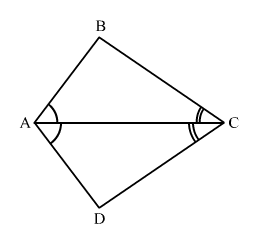# ABCD is a quadrilateral such that diagonal AC bisects the angles ∠A and ∠C.

Question:

ABCD is a quadrilateral such that diagonal AC bisects the angles ∠A and ∠C. Prove that AB AD and CB CD.

Solution:Given: In quadrilateral ABCDAC bisects the angles ∠A and ∠C.

To prove: AB AD and CB CD

Proof: